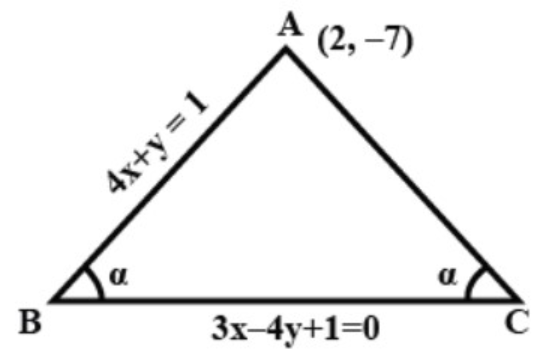A line 4x+y=1 through the point A (2,−7) meets the line BC whose equation is 3x−4y+1=0 at the point B. Find the equation to the line AC so that -AB=AC.Verified
147k+ views
Hint:Here we will find the slope of the first line and second line initially and then with the help of the slopes found and given condition we will find the slope of the given line. With the slope found and the given points we will find the equation of the new line.

Formula used:$y{\rm{ }} = {\rm{ }}mx + b$ where$m$ in the equation is the slope of the line
We know that the formula
$\theta = {\tan ^{ - 1}}\left| {\dfrac{{{m_1} - m}}{{1 + {m_1}m}}} \right|$
Where m is the slope of the line.

Here it is given that
AB is line with equation 4x + y = 1
Let us now compare it with the equation $y{\rm{ }} = {\rm{ }}mx + b$ where $m$ in the equation is the slope of the line, we get,
The slope of the line is $- 4$
Now let us consider the line BC given by the equation 3x - 4y + 1 =0
Let us rewrite the equation as follows $y = \dfrac{3}{4}x + \dfrac{1}{4}$
By comparing with the standard equation $y{\rm{ }} = {\rm{ }}mx + b$ where $m$ in the equation is the slope of the line, we get,
The slope of the line as $\dfrac{3}{4}$Here we have to find the equation to the line AC. If m be the slope of AC, the AB and AC are equally inclined to BC.
We know that the formula
$\theta = {\tan ^{ - 1}}\left| {\dfrac{{{m_1} - m}}{{1 + {m_1}m}}} \right|$
For line AB and BC, we have
Let $${m_1} = \dfrac{3}{4}\,\&\, m = - 4$$ be the slopes of AC and AB respectively.
$\alpha = {\tan ^{ - 1}}\left| {\dfrac{{\dfrac{3}{4} - \left( { - 4} \right)}}{{1 + \left( {\dfrac{3}{4}} \right)\left( { - 4} \right)}}} \right|$
Let us operate by tan on both sides we get,
${\rm{tan}}\alpha = \left| {\dfrac{{\dfrac{3}{4} - \left( { - 4} \right)}}{{1 + \left( {\dfrac{3}{4}} \right)\left( { - 4} \right)}}} \right|$
And for line AC and BC, we have
Let ${m_1} = \dfrac{3}{4}$ be the slope of AC and m be the slope of BC.
$\theta = {\tan ^{ - 1}}\left| {\dfrac{{\dfrac{3}{4} - m}}{{1 + \left( {\dfrac{3}{4}} \right)m}}} \right|$
Let us operate by tan on both sides of the equation we have,
${\rm{tan}}\theta = \left| {\dfrac{{\dfrac{3}{4} - m}}{{1 + \left( {\dfrac{3}{4}} \right)m}}} \right|$
Where m is the slope of line AC.
According to question, we are given that $- AB = AC$
So, we have the slope of the lines as follows
-slope of the line AB= slope of the line BC.
That is $- \tan \alpha = \tan \theta$
On substituting the values we get,
$- \dfrac{{\dfrac{3}{4} - \left( { - 4} \right)}}{{1 + \left( {\dfrac{3}{4}} \right)\left( { - 4} \right)}} = \dfrac{{\dfrac{3}{4} - m}}{{1 + \left( {\dfrac{3}{4}} \right)m}}$
Let us now simplify the above equation we get,
$\dfrac{{ - 19}}{{ - 8}} = \dfrac{{3 - 4m}}{{3m + 4}}$
$\Rightarrow 19\left( {3m + 4} \right) = 8\left( {3 - 4m} \right)$
By solving the above equation we get,
$57m + 76 = - 32m + 24$
Then the value of m is
$m = \dfrac{{ - 52}}{{89}}$
Also the line passes through the point A (2, -7),
Using the slope and passing points we get the equation of line using the following formula,
Formula of equation of line is $y - {y_1} = m\left( {x - {x_1}} \right)$
By substituting the known values we get,
$y + 7 = - \dfrac{{52}}{{89}}\left( {x - 2} \right)$
By multiplying both the sides by 89 and solving the equation we get,
The equation of the line AC is $89y + 52x + 519 = 0$
Hence, the equation to the line AC is ${\rm{52x}} + {\rm{89y}} + {\rm{519}} = 0$

Additional Information:The formula $y - {y_1} = m\left( {x - {x_1}} \right)$ is usually described as the 'point-slope form' for the equation of a line. It is useful because if you know one point on a certain line and the slope of that certain line, then you can define the line with this type of formula and, thus, find all the other points on that certain line.

Note:Here while finding the slope of BC we must use the relation between slope and tangent of the line. Also we use the slope and intersection points to find the equation of line.